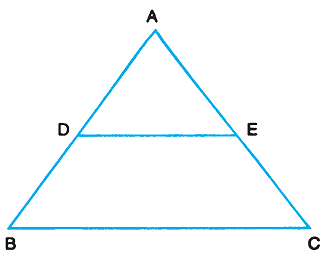# Properties of Triangle

Triangle properties are divided into: (i) Angle Properties (ii) Side Angle Properties.

### Angle Properties

Angle Sum Property of a Triangle

The sum of the three angles of triangle is 180°.

∠A + ∠B + ∠C = 180°

Exterior Angles of a Triangle

The angle formed by a side of the triangle produced and another side of the triangle is called an exterior angle of the triangle. Corresponding to an exterior angle of a triangle, there are two interior opposite angles. Interior opposite angles are the angles of the triangle not forming a linear pair with the given exterior angle.An exterior angle of a triangle is equal to the sum of the two interior opposite angles.

∠ACD = ∠A + ∠B

### Side Angle Relationship

Equal Angles and Equal Sides

The angles opposite to equal sides of a triangle are equal. Conversely, the sides opposite to equal angles of a triangle are equal.

Perpendiculars to Equal Sides

Perpendiculars (altitudes) drawn to equal sides, from opposite vertices of an isosceles triangle are equal. The result can be extended to an equilateral triangle - all the three altitudes of an equilateral triangle are equal.

### Inequalities in Triangle

If two sides of a triangle are unequal, then the longer side has the greater angle opposite to it. In a triangle, the greater angle has longer side opposite to it.

Sum of any two sides of a triangle is greater than the third side.

### Mid Point Theorem

In a triangle the line segment joining the mid points of any two sides is parallel to the third side and is half of it. Conversely, the line drawn through the mid point of one side of a triangle parallel to the another side bisects the third side.### Similarity of Triangles

If a perpendicular is drawn from the vertex of the right angle of a right triangle to the hypotenuse, the triangles on each side of the perpendicular are similar to each other and to the original triangle.

The ratio of the areas of two similar triangles is equal to the ratio of the squares of their corresponding sides.

Pythagoras Theorem: In a right triangle, the square on the hypotenuse is equal to sum of the squares on the other two sides.